Question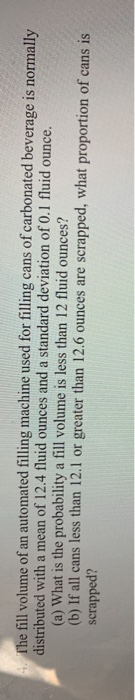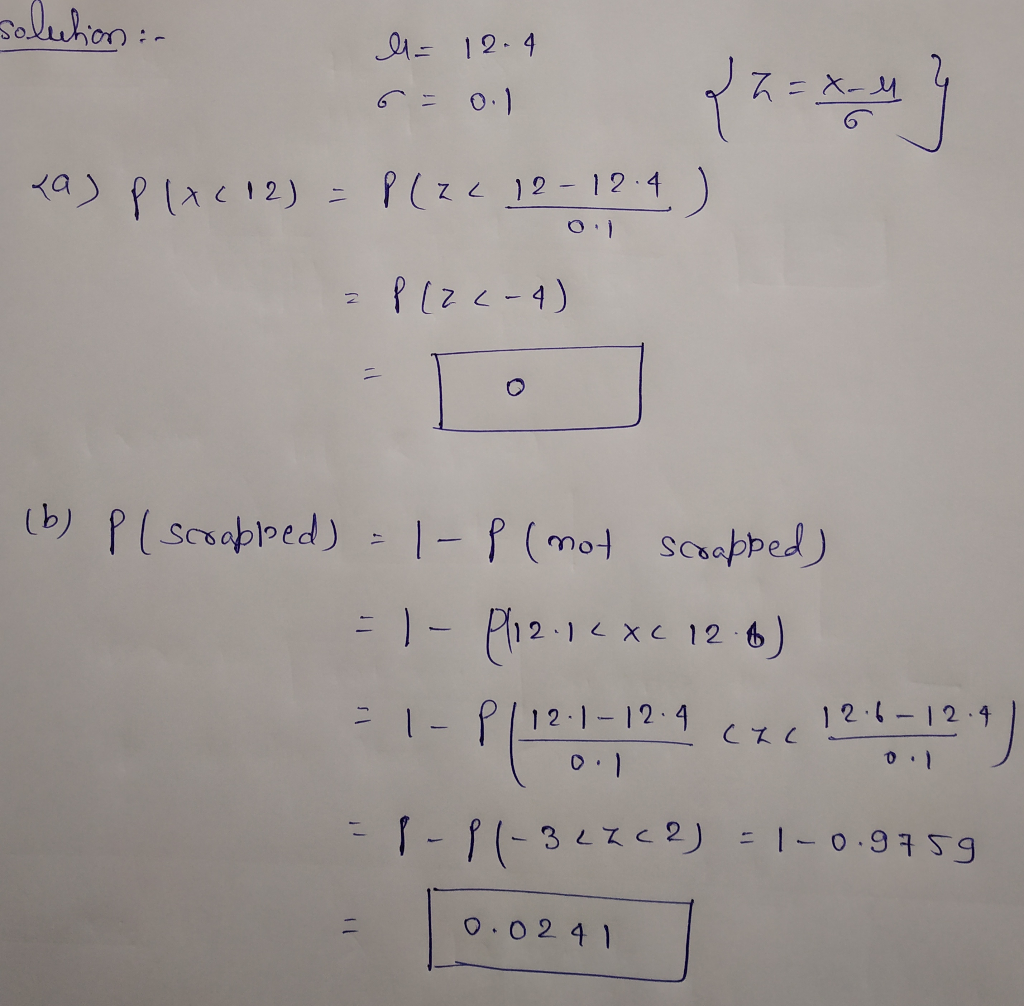#### Earn Coins

Coins can be redeemed for fabulous gifts.

Similar Homework Help Questions
• ### 3. The fill volume of an automated filling machine used for filling cans of carbonated beverage...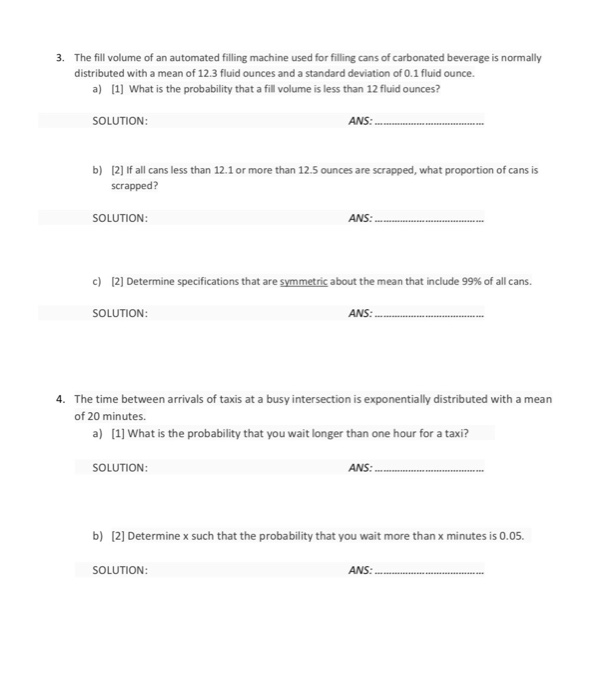3. The fill volume of an automated filling machine used for filling cans of carbonated beverage is normally distributed with a mean of 12.3 fluid ounces and a standard deviation of 0.1 fluid ounce. a) 1] What is the probability that a fill volume is less than 12 fluid ounces? SOLUTION ANS: b) 2] If all cans less than 12.1 or more than 12.5 ounces are scrapped, what proportion of cans is scrapped? SOLUTION: ANS: c)  Determine specifications that...

• ### Soft drink cans are filled by an automated filling machine and the standard deviation is 0.5...

Soft drink cans are filled by an automated filling machine and the standard deviation is 0.5 fluid ounce. Assume that the fill volumes of the cans are independent, normal random variables. Round your answers to four decimal places (e.g. 98.7654). What is the standard deviation of the average fill volume of 100 cans? If the mean fill volume 12.1 ounces, what is the probability that the average fill volume of the 100 cans is less than 12 fluid ounces? What...

• ### Soft drink cans are filled by an automated filling machine and the standard deviation is 0.5...

Soft drink cans are filled by an automated filling machine and the standard deviation is 0.5 fluid ounce. Assume that the fill volumes of the cans are independent, normal random variables. Round your answers to four decimal places (e.g. 98.7654). If the mean fill volume is 12.1 fluid ounces, what should the standard deviation of fill volume equal so that the probability that the average of 100 cans is less than 12 fluid ounces is 0.005?

• ### Soda six-packs Most soda cans list the volume of soda as 12 fluid ounces. As with...

Soda six-packs Most soda cans list the volume of soda as 12 fluid ounces. As with all process, some variation occurs when filling soda cans. Suppose that a company knows this and tries to over-fill cans a bit, so that the actual volume of soda in a can follows a normal distribution with mean 12.1 fluid ounces and standard deviation .15 fluid ounces. a) What proportion of soda cans filled by this process will contain less than 12 fluid ounces?...

• ### A postmix beverage machine is adjusted to release a certain amount of syrup into a chamber...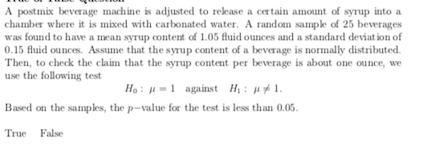A postmix beverage machine is adjusted to release a certain amount of syrup into a chamber where it is mixed with carbonated water. A random sample of 25 beverages was found to have a mean syrup content of 1.05 fluid Ounces and a standard deviation of 0.15 fluid Ounces. Assume that the syrup content of a beverage is normally distributed. Then, to check the claim that the syrup content per beverage is about one ounce, we use the following test...

• ### True or False Question A postmix beverage machine is adjusted to release a certain amount of...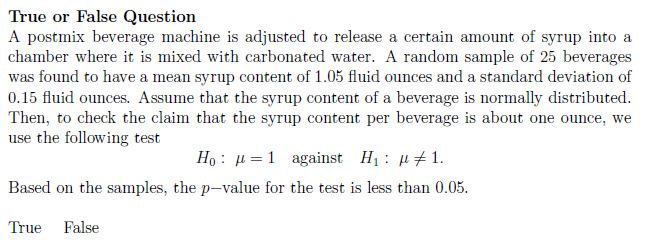True or False Question A postmix beverage machine is adjusted to release a certain amount of syrup into a chamber where it is mixed with carbonated water. A random sample of 25 beverages was found to have a mean syrup content of 1.05 fluid ounces and a standard deviation of 0.15 fluid Ounces. Assume that the syrup content of a beverage is normally distributed. Then, to check the claim that the syrup content per beverage is about one ounce, we...

• ### The amounts a soft drink machine is designed to dispense for each drink are normally​ distributed,...

The amounts a soft drink machine is designed to dispense for each drink are normally​ distributed, with a mean of 12.1 fluid ounces and a standard deviation of 0.2 fluid ounce. A drink is randomly selected. ​(a) Find the probability that the drink is less than 11.9 fluid ounces. ​(b) Find the probability that the drink is between 11.7 and 11.9 fluid ounces. ​(c) Find the probability that the drink is more than 12.4 fluid ounces. Can this be considered...

• ### True or False Question A postmix beverage machine is adjusted to release a certain amount of...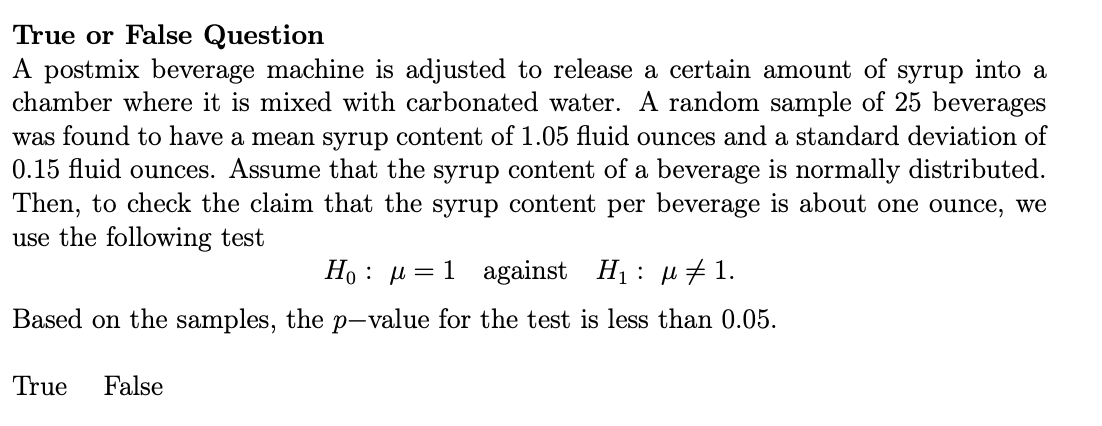True or False Question A postmix beverage machine is adjusted to release a certain amount of syrup into a chamber where it is mixed with carbonated water. A random sample of 25 beverages was found to have a mean syrup content of 1.05 fluid ounces and a standard deviation of 0.15 fluid ounces. Assume that the syrup content of a beverage is normally distributed. Then, to check the claim that the syrup content per beverage is about one ounce, we...

• ### True or False Question A postmix beverage machine is adjusted to release a certain amount of...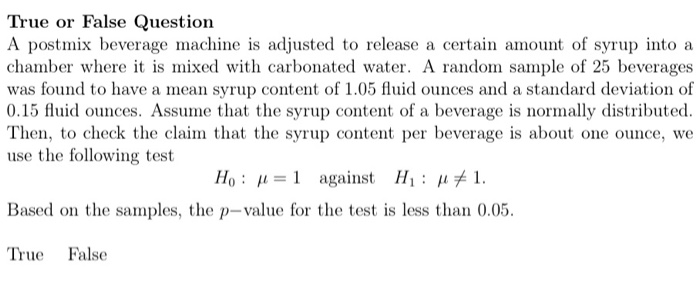True or False Question A postmix beverage machine is adjusted to release a certain amount of syrup into a chamber where it is mixed with carbonated water. A random sample of 25 beverages was found to have a mean syrup content of 1.05 fluid ounces and a standard deviation of 0.15 fluid ounces. Assume that the syrup content of a beverage is normally distributed. Then, to check the claim that the syrup content per beverage is about one ounce, we...

• ### Orange 52. Juice Dispensing Machine A beverage company uses a machine to fill half-gallon bottles with...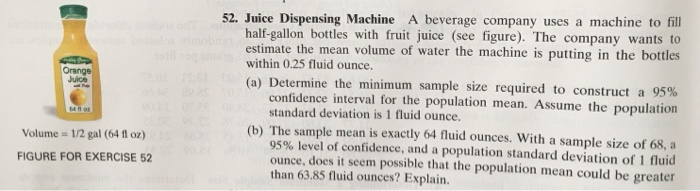Orange 52. Juice Dispensing Machine A beverage company uses a machine to fill half-gallon bottles with fruit juice (see figure). The company wants to estimate the mean volume of water the machine is putting in the bottles within 0.25 fluid Ounce. (a) Determine the minimum sample size required to construct a 95% confidence interval for the population mean. Assume the population standard deviation is 1 fluid Ounce. (b) The sample mean is exactly 64 fluid ounces. With a sample size...